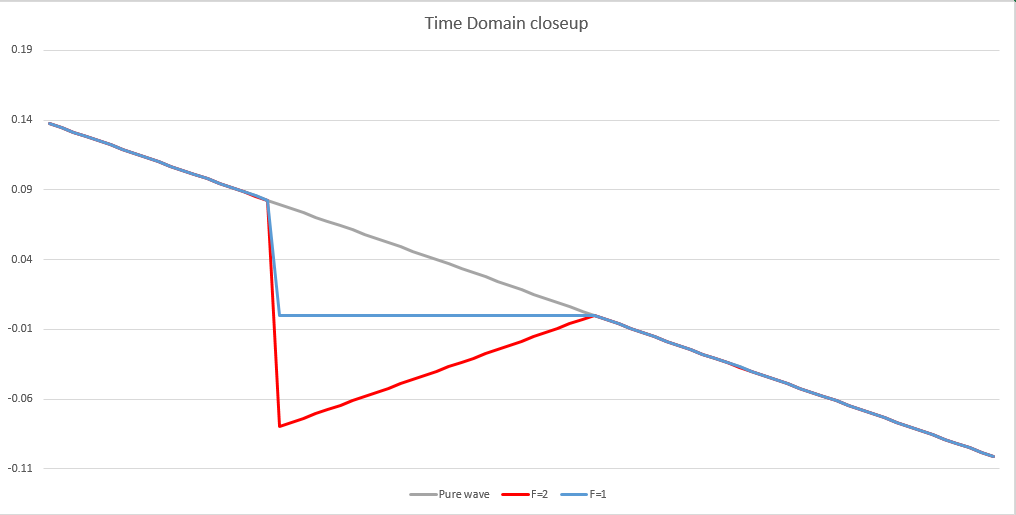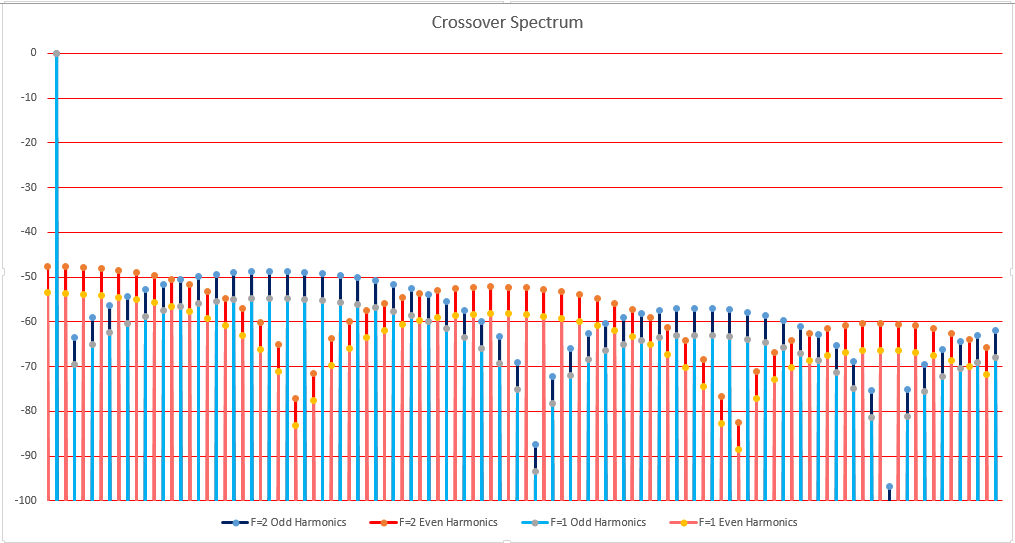Crossover distortion comparison

# Harmonics=Area: It's the LAW!

Published on December 16, 2018

I've been having fun doing experiments with inverse FFTs controlling the time domain waveform. I know, it's a sickness. While my coworkers were surfing the web for porn or looking for a new job, I was filtering waveforms in Frequency Domain by manipulating their spectra. Back then I was using Credence's AWT or Nextest's MSWT, but now I use Excel and I finally found a really simple example to prove the Bullard Laws of Harmonics #1, "Harmonic amplitudes are proportional to the area of the distortion." Let me show you.

In the previous article I clipped the peak and then manipulated the spectrum to change the Time Domain waveform. This time I woke up at 2AM and thought I should try simulating Crossover Distortion the way I do in Chapter 4 of Distortion. Crossover is very common in Class B and Class AB amplifiers, but generally it's symmetrical. However, to understand how it works, I like to start with asymmetrical distortion which gives me both Odd (blue) and Even (red) harmonics. One nice thing about simulating Crossover distortion is that everything is very linear at the zero crossing, unlike the peak where things get a little strange because of the curvature of the peak.Notice that the red triangle (labeled F=2) is twice the area of the blue triangle formed by the simple crossover distortion (labeled F=1). Since I doubled the amplitudes of all the harmonics, both the ones I could see and the ones I can't see (the aliases) it should, according to my theory, double the area of the distortion, which it did! Now to make absolutely sure, I then did an FFT on the distorted waves, the simple crossover distortion (F=1) and the doubled distortion (F=2).I kept my color code, as best as I could given that I have four kinds of spectral bins to show you. The Even bins are color coded red (two shades) and the Odd harmonics are color coded blue (two shades). Since I used a punctuated line graph, you can see where each bin starts. The take away here is that the F=2 bins, both Odd and Even, are 6.02dB higher than the F=1 (simple crossover distortion) bins. Double the area, double the harmonic energy, once again proving that my books are right. The Bullard Laws of Harmonics, Law #1 says that harmonic amplitudes are proportional to the area of the distortion. I can't think of any way to prove it with more clarity than the graphs you see above.I kept my color code, as best as I could given that I have four kinds of spectral bins to show you. The Even bins are color coded red (two shades) and the Odd harmonics are color coded blue (two shades). Since I used a punctuated line graph, you can see where each bin starts. The take away here is that the F=2 bins, both Odd and Even are 6.02dB higher than the F=1 (simple crossover distortion) Odd and Even bins. Double the area, double the harmonic energy, once again proving that my books are right. The Bullard Laws of Harmonics, Law #1 says that harmonic amplitudes are proportional to the area of the distortion. I can't think of any way to prove it with more clarity than the graphs you see above.

Now, why it took 70 years for someone to figure this out is beyond me. And why it had to be me both thrills me, and worries me. I don't have an advanced degree, I wasn't trained for this stuff. Tube testers was as far as my training went back in college. I got an A on that paper (and everyone in the class copied it) but little did I know then that I would be the guy who discovered how harmonics work. It could have been any of you, but it wasn't, it was me. And boy, I wish my dad had lived to see this day!